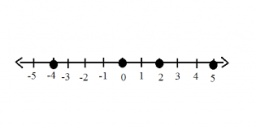# Calculate 6729

Calculate the larger of the two numbers if the smaller number is the third of the larger number and their sum is 48.

x =  36

### Step-by-step explanation:

x+y= 48
y = x/3

x+y = 48
x-3y = 0

Row 2 - Row 1 → Row 2
x+y = 48
-4y = -48

y = -48/-4 = 12
x = 48-y = 48-12 = 36

x = 36
y = 12

Our linear equations calculator calculates it.Did you find an error or inaccuracy? Feel free to write us. Thank you!

Tips for related online calculators
Do you have a linear equation or system of equations and looking for its solution? Or do you have a quadratic equation?